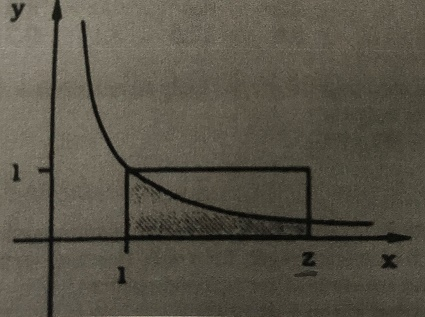# The figure below shows the graph y = \frac{1}{x} for x > 0. A rectangle with one side along the...

## Question:

The figure below shows the graph {eq}y = \frac{1}{x} {/eq} for x > 0. A rectangle with one side along the x-axis has the upper left corner of the point (1, 1) and the lower right corner of the point (z, 0).

Determine z with four digging digits, if the shaded area is half of the rectangle's area.## Area Under Curve

In calculus, we learn that we can determine the area under a curve through the use of a definite integral. If given an interval (a,b), then the area under the curve, f(x), is given by the following

{eq}\int_a^b {f(x) dx} {/eq}

We will use this idea to solve our problem.

We know that the area of half a rectangle is given as

{eq}A = \frac{l\times w}{2} {/eq}

Here length is z and width is 1, so we have

{eq}A = \frac{1 \times (z-1)}{2} = \frac{z-1}{2} {/eq}

We can solve as follows

{eq}\int_1^Z {\frac{1}{x} dx} {/eq}

{eq}\rightarrow ln(x) |_1^z = ln(z) - ln(1) = ln(z) {/eq}

So we can solve for z as follows

{eq}ln(z) = \frac{z-1}{2} {/eq}

{eq}\rightarrow z= 3.5129 {/eq}